﻿ Segmentation Model for Noisy and Intensity Inhomogeneity Images via Logarithmic Density Function

# Segmentation Model for Noisy and Intensity Inhomogeneity Images via Logarithmic Density Function

Ali S* and Dayyan B
Department of Mathematics, University of Peshawar, Old Jamrud Rd, Peshawar, Pakistan
*Corresponding Author: Ali S, Department of Mathematics, University of Peshawar, Old Jamrud Rd, Peshawar, Pakistan, Tel: +92 91 9216701, Email: [email protected]

Received Date: Dec 22, 2017 / Accepted Date: Jan 11, 2018 / Published Date: Jan 18, 2018

### Abstract

This manuscript is devoted to the study of a new image segmentation model for noisy and intensity inhomogeneity images based on logarithmic density function. Local image information is necessary for inhomogeneous images but at the same time, it is defective for noisy images as a consequence local information misguide the motion of active contour. However, the logarithmic function in our new proposed model is capable to capture minute details in images, while ignoring the noise in it which makes it robust in such kinds of images. Comparing with local Chan-Vese Model our new proposed model gives better performance treating noisy and intensity inhomogeneity images. Finally, experiments on some noisy and intensity inhomogeneity images show the robustness of our new proposed model.

Keywords: Segmentation; Level set; LCV model; Statistical model; Gaussian distribution

#### INSPEC Classification

A4230V, B6135, C1250M, C5260B

#### Introduction

The segmentation of image is one of the demanding task in the field of image processing and computer vision field, due to the applications of such fields which are ranging from engineering sciences to medical sciences . Its objective is to divide an image in different parts to make it meaningful and easier to analyze, for detail study see monographs such as [2-9]. Till now, many kinds of algorithm have been suggested to find the answer to the image segmentation problem. Also several approaches are used to enhance the outcome of image segmentation algorithm [10-12]. Among these, LCV model is of a great importance, which use both local and global image-information for segmentation of image. The LCV model, by performing few iterations used local image details to segment the images with intensity inhomogeneity, efficiently. Furthermore, the re-initialization step vastly used in traditional level set approaches which consume to much of time, can be shunned by the introduction of a newly penalizing energy regarding regularization term. Due to this, the consumption of time is lessened [13-19]. Especially, level set evolution procedure having the evolution curve, which can automatically ends on exact boundaries/edges of the given objects. However, in some complex noisy images it nearly fails to tackle the segmentation, by seizing the noise in the image to use the local image information. At the moment, it is also of great importance to deal with the problem of segmentation in noisy images, because the real world that we get have noise.

Motivated by all above, the given monograph deals with a new noisy image segmentation model, which provide better and more efficient results when apply on noisy images [20-24]. This new propose model can capture more information regarding the given noisy image, avoiding the noise also at the same time in the final segmented result. On the other hand, traditional models can segment noisy image but these models also captured the noise at the same time, which is defective and not providing good result. For detail study regarding traditional image segmentation models with different approaches, see the monographs such as [7-11,20]. The idea about our new propose model is nothing but it may be the combination of ideas regarding the local Chan-Vese model and non-linear diffusion model which is based on noisy image segmentation. The proposed model consider the region information sufficiently. It is not necessary to consider the re-initialization of the curve, due to the application of Gaussian density function, implies that it must be noise robust. This is because that we consider the mean as well as global variance of the given image. Compared with classical LCV model, our new propose model for noisy image segmentation is much better and reduce the number of noise in the final segmented image result. The above mention edge of our new propose model can be seen through experimental results, which were presented in the last section of this monograph.

#### Previous Works

In this section, we discuss some previous works regarding noisy image segmentation and intensity inhomogeneity image segmentation. In more precise way, we can briefly analyze two models, which are the following:

Nonlinear diffusion equation model for noisy image segmentation

Local Chen-Vese (LCV) model

Taking in account, the main ideas and limitations regarding above mentioned models, we propose our new model in section 3 which covers some limitations of LCV model, i.e., as we know that LCV model is not capable of giving efficient and good result in noisy intensity inhomogeneity images but our propose model is better in such type of image segmentations. The robustness of our new proposed model is shown through some experimental results, in section 4 of this monograph. The last section of this monograph is devoted to the concluding note about our new segmentation model.

Noisy image segmentation based on nonlinear diffusion equation (NSDB) model

The NSDB model  used statistical information in order to reduce the noise in segmentation. In NSBD model the infimum variance term in FEI model is replaced by a statistical term, which is being consider as a part regarding external energy at first.

Generally the above model is given as: arg(minCE(C)), where E(C) is the energy functional of this model, which has the following form: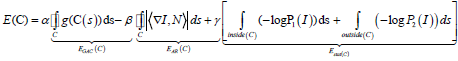In above energy term, I(u,v) represents a gray level image, ∇I(u,v)={Iu,Iv} denotes the gradient vector of the given image. The notation C: [0,L] 7↦R2 is used for a parametric curve, and α,β,γ are any positive constants. Let us consider C(s)={u(s),v(s)}, where s denotes arc length parameter. The notation Cs={us,vs} used for tangent vector of a curve, implies that the associated normal direction is given as N(s)={− vs,us}. The model in discussion, i.e., NSDB model, consolidate many geometric measures to a unified variational framework and obtain the required statistical active contour model. In the Gaussian density case, we have two parameter’s only, i.e., mean m and variance σ2 should be obtained. Let us consider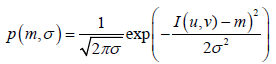then the statistical active contour model is rearranged as below: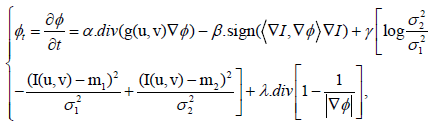Where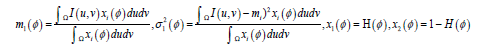and

H(p)=1, if p>0

0, if p<0

is a Heaviside function.

Local Chan-Vese (LCV) model

Many traditional segmentation model faces the problem of inhomogeneity segmentation, which happen in many real world images. To overcome the problem of inhomogeneous intensity segmentation, the LCV model combine both the local and global statistical information. The general form of overall energy functional of LCV model is:

F=αFG+βFL+FR,

where FG, FL and FR are global, local and regularized terms respectively and defined as: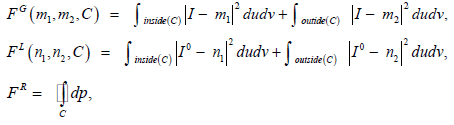where C is a closed curve: m1, m2, n1 and n2 are constants inside and outside C and I’ is a smooth image define as I’=gkII and gk is an average operator with k × k convolution size window. Now the overall energy functional of LCV model in regularized form can be formulated as: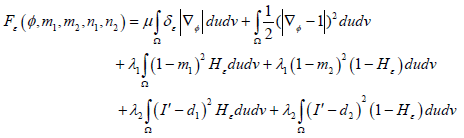The initial term reveal the regularized term, which is used for smoothing purpose of contour and also this term is used to make the contour tight. While the next two terms in above equation represents the local and global terms, which came in application for capturing local and global information regarding the given image. Minimization of Fε(φ,m1,m2,n1,n2) with respect to m1, m2, n1 and n2 yields the following: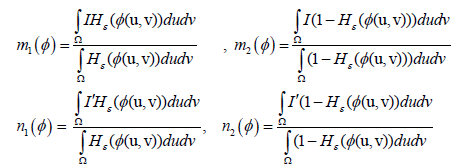By minimizing Fε with respect to φ, the Euler-Lagrange’s equation for φ is given by: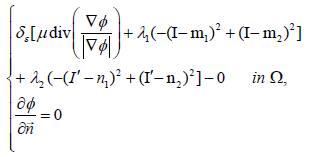The below equation is consider for solution.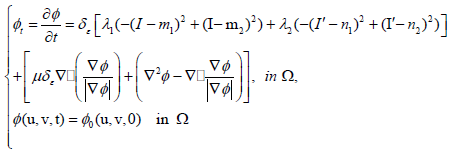Thus LCV model encompass the inhomogeneity problems. In fact, LCV model has also some drawbacks, because it fails in images which has low contrast, images regarding low frequencies, unilluminated objects, overlapping regions of homogeneous intensities, in such situations the results of the LCV model is not to much satisfactory.

#### Our Proposed Local Chan-Vese Noisy Image Segmentation Model

This section deals with the study of our new proposed SEGMENTATION model. It is known that LCV model perform better in intensity inhomogeneity images but fails in noisy image segmentation. Since, LCV model uses local image information due to which it sometimes almost fails to segment some sort of noisy images. Hence, to reduce that mention limitations regarding LCV model, we proposed a new model in the following way.

For our new proposed model, we define a new logarithmic density function and also use few statistical information to reduce the problem regarding image segmentation in such type of noisy images. The energy functional of our new proposed model is given by: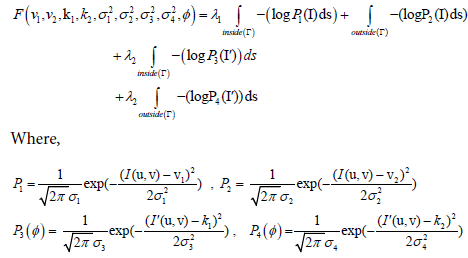and ν1, ν2 are the averages of given image inside and outside respectively and d1, d2 are the averages of difference image inside and outside respectively and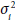, where i=1,2,3,4 denotes the corresponding variances.

Now we minimizing the energy functional. For a fixed level set function φ, we minimize the energy functional with respect to ν1, ν2, κ1, κ2 and variances. Using variational calculus, we can prove that the constant functions ν1, ν2, κ1, κ2 and variances that reduce the energy functional for a fixed function φ as given below: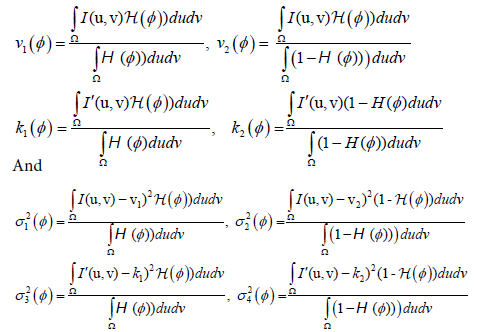Now keeping ν1, ν2, κ1, κ2 and variances fixed, and minimizing the overall energy functional (3.1) with respect to φ. We did the minimization by involving a time artificial variable t ≥ 0, hence minimization of energy functional for fixed ν1, ν2, κ1, κ2 and variances is given by: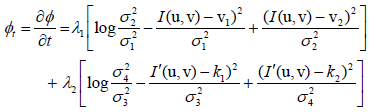The following section of this monograph, shows the applicability of the above new research work. In precise way we can say that the coming section shows the numerical work regarding the energy functional of our new proposed model.

#### Experimental Results

This section contains some experimental results of our new proposed model. These experimental results show the efficiency and better performance of our new proposed model, as compared to LCV model regarding noisy images. Initial contour can be considered anywhere on the original noisy image. For simplicity we denote by:

the LCV model and the proposed SEGMENTATION model.

Experimental result of LCV model

The figure illustrating the performance of LCV model. (a) Original synthetic Image (b) LCV model Result (c) Final Result (Figure 1).

Figure 1: The figure illustrating the performance of LCV model. (a) Original synthetic Image (b) LCV model Result (c) Final Result.

Experimental result of proposed model

The figure illustrating the performance of our new proposed SEGMENTATION model. (a) Original synthetic Image (b) proposed model Result (c) Final Result (Figure 2).

Figure 2: The figure illustrating the performance of our new proposed SEGMENTATION model. (a) Original synthetic Image (b) proposed model Result (c) Final Result.

Remark

The above figure is an original satellite image. The result of Figures 1 and 2 shows the robustness of LCV and proposed models, respectively. Clearly, the proposed model Result is more efficient as compared to LCV model, because proposed model captured more information of the original image.

Experimental result of LCV model

The figure illustrating the performance of LCV model. (a) Original synthetic Image (b) LCV model Result (c) Final Result (Figure 3).

Figure 3: The figure illustrating the performance of LCV model. (a) Original synthetic Image (b) LCV model Result (c) Final Result.

Experimental result of proposed model

The figure illustrating the performance our proposed SEGMENTATION model. (a) Original synthetic Image (b) proposed model Result (c) Final Result (Figure 4).

Figure 4: The figure illustrating the performance our proposed SEGMENTATION model. (a) Original synthetic Image (b) proposed model Result (c) Final Result.

Remark

The above figure is an original satellite image. The result of Figures 3 and 4 shows the robustness of LCV and proposed models, respectively. Clearly, the result of proposed model is more efficient as compared to LCV model, because proposed model captured more information of the original image.

Experimental result of LCV mode

The figure illustrating the performance of LCV model. (a) Original Image (b) LCV model Result (c) Final Result (Figure 5).

Figure 5: The figure illustrating the performance of LCV model. (a) Original Image (b) LCV model Result (c) Final Result.

Experimental result of proposed model

The figure illustrating the performance our proposed SEGMENTATION model. (a) Original Image (b) proposed model Result (c) Final Result (Figure 6).

Figure 6: The figure illustrating the performance our proposed SEGMENTATION model. (a) Original Image (b) proposed model Result (c) Final Result.

Remark

The above figure displays a real noisy medical image. The result of Figures 5 and 6 shows the robustness of LCV and proposed models, respectively. Clearly, the result of proposed model is more efficient as compared to LCV model, because proposed model captured less noise.

Experimental result of LCV model

The figure illustrating the performance LCV model. (a) Original Image (b) LCV model Result (c) Final Result (Figure 7).

Figure 7: The figure illustrating the performance LCV model. (a) Original Image (b) LCV model Result (c) Final Result.

Experimental result of proposed model

The figure illustrating the performance our proposed SEGMENTATION model. (a) Original Image (b) proposed model Result (c) Final Result (Figure 8).

Figure 8: The figure illustrating the performance our proposed SEGMENTATION model. (a) Original Image (b) proposed model Result (c) Final Result.

Remark

The above figure is a real medical noisy image. The result of Figures 7 and 8 shows the robustness of LCV and proposed models, respectively. Clearly, the result of proposed model is more efficient as compared to LCV model, because proposed model captured less noise.

Experimental result of LCV mode

The figure illustrating the performance of LCV model. (a) Original Image (b) LCV model Result (c) Final Result (Figure 9).

Figure 9: The figure illustrating the performance of LCV model. (a) Original Image (b) LCV model Result (c) Final Result.

#### Experimental Result of Proposed Model

The figure illustrating the performance our proposed SEGMENTATION model. (a) Original Image (b) proposed model Result (c) Final Result (Figure 10).

Figure 10: The figure illustrating the performance our proposed SEGMENTATION model. (a) Original Image (b) proposed model Result (c) Final Result.

Remark

The above figure is an original satellite moon image. The result of Figures 9 and 10 shows the robustness of LCV and proposed models, respectively. Clearly, the result of proposed model is more efficient as compared to LCV model, because proposed model captured more information of the original image.

Experimental result of LCV model

The figure illustrating the performance of LCV model. (a) Original Image (b) LCV model Result (c) Final Result (Figure 11).

Figure 11: The figure illustrating the performance of LCV model. (a) Original Image (b) LCV model Result (c) Final Result.

Experimental result of proposed model

The figure illustrating the performance our proposed model. (a) Original Image (b) proposed model Result (c) Final Result (Figure 12).

Figure 12: The figure illustrating the performance our proposed model. (a) Original Image (b) proposed model Result (c) Final Result.

Remark

The above figure is an original satellite image. The result of Figures 11 and 12 shows the robustness of LCV and proposed models, respectively. Clearly, the result of proposed model is more efficient as compared to LCV model, because proposed model captured more information of the original image.

#### Conclusion

In concluding note, precisely we can say that our new proposed model for noisy intensity inhomogeneity image segmentation is nothing but actually the combination regarding local Chan-Vese (LCV) model and Noisy image segmentation based on nonlinear diffusion model .

#### Competing Interest

Authors announced that this research work has no competing interest.

#### References

Citation: Ali S, Dayyan B (2018) Segmentation Model for Noisy and Intensity Inhomogeneity Images via Logarithmic Density Function. J Appl Computat Math 7: 388. DOI: 10.4172/2168-9679.1000388

Copyright: ©2018 Ali S, et al. This is an open-access article distributed under the terms of the Creative Commons Attribution License, which permits unrestricted use, distribution, and reproduction in any medium, provided the original author and source are credited.

Select your language of interest to view the total content in your interested language

##### Recommended Journals
Viewmore
###### Article Usage
• Total views: 1298
• [From(publication date): 0-2018 - Dec 16, 2019]
• Breakdown by view type
• HTML page views: 1252Can't read the image? click here to refresh